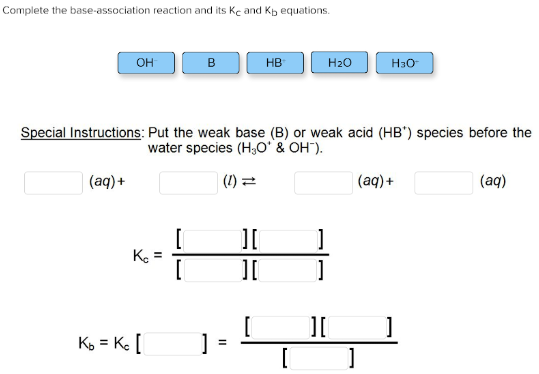# Complete the base-association reaction and its Kc and Kb equations. OH- B HB- H2O H3O- Special instructions: Put the weak base (B) or weak acid (HB+) species before the water species (H3O+ & OH-). ___(aq) + ___(l) <-> ___(aq) + ___(aq) Kc = [__][__]/[__][__] Kb = Kc [__] = [__][__]/[__]